#### Email Us

Follows

The ability to lookup number values in excel is a must-have for all data analysts. Some Excel users just know the basic VLOOKUP function but there are 7 more functions to lookup values.

The more lookup function you know the better you become in retrieving numbers like sales for a certain month, a balance for a customer, the price of a product, production for a certain day, etc.

As long as the return value is numeric any of these formulas will work.

Here are the 7+ ways to lookup numerical values:

1. SUMPRODUCT
2. SUMIF/SUMIFS/SUM
3. AGGREGATE
4. LOOKUP
5. INDEX & MATCH
6. MAX or MAXA
7. VLOOKUP
8. DATABASE FUNCTIONS i.e DSUM,DMAX, DMIN, DGET, DAVERAGE, DPRODUCT
9. USE OF SLICERS
10. OTHER NUMEROUS

For example, using below data lookup sales values for the month of August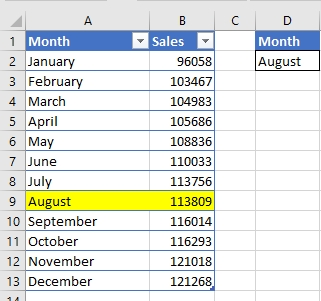# SUMPRODUCT

• Syntax = SUMPRODUCT(Array1,Array2,…………,Array30)
• Multiplies parallel values in matching arrays and returns the sum of their products.
• Arrays must be of equal size and cannot be a mix of columns and rows
• Treats non-numeric values as zero

How it works

`=SUMPRODUCT((A2:A13=D2)*(B2:B13))`

►(A2: A13=D2) Returns an array of TRUE/FALSE which SUMPRODUCT converts into 1/0

► (B2: B13)  Returns an array of sales values

The sum of the product of the above two arrays equals  sales for the criteria month

# SUMIF

• Syntax =SUMIF(criteria range, criteria, [sum range])
• Sums values in a range that meet a certain criterion
• It can only sum a range that is up to 255 characters
• It accepts Wildcards in the criterion argument
• Sum range is optional, thus, when omitted SUMIF sums the criteria range

How It Works

`=SUMIF(A2:A13,D2,B2:B10)`

►A2: A13 represents our Months criteria range

►D2 represents our criterion month (August)

►B2: B10 represents our sales values to lookup

# AGGREGATE FUNCTION

• Can either be in a Reference form or Array form

The syntax for Reference;

=AGGREGATE(function_num, options, ref1, [ref2], …)

The syntax for Array;

=AGGREGATE(function_num, options, array, [k])

Function_num a number between 1 and 19 that determines which function to use.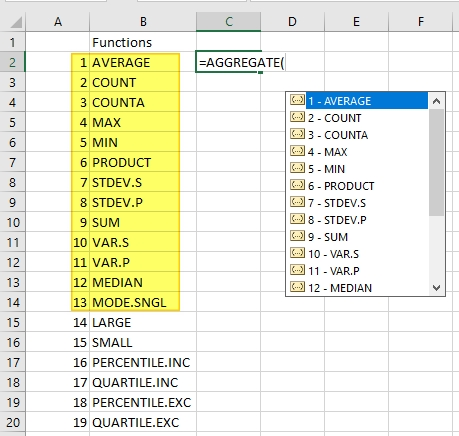Function 1 to 13 use reference form

While Functions 14 to 19 use array form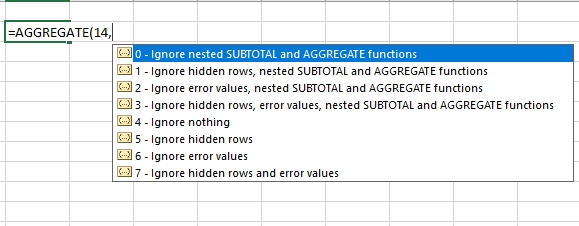Options a number between 0 and 7 that determines which values to ignore during an evaluation

NB:

• It returns a #VALUE! Error if a required 2nd Ref argument is not provided
• Works well with data in columns or in vertical ranges

How It Works

`=AGGREGATE(14,7,B2:B13/(A2:A13=D2),1)`
• We are using an array function (number 14—LARGE) and Option 7 which ignores hidden rows and error values
• B2: B13 returns our sales values in an array
• (A2: A13=D2) Returns array of TRUE/FALSE which AGGREGATE converts into 1/0
• By dividing our sales values array (B2: B13) with an array of 1/0 we get an array of errors (where the divisor is zero) and Sales value (where the divisor is 1)
```{#DIV/0!;#DIV/0!;#DIV/0!;#DIV/0!;#DIV/0!;#DIV/0!;#DIV/0!
;113809;#DIV/0!;#DIV/0!;#DIV/0!;#DIV/0!}```
• Since we choose Option 7 which ignores hidden rows and error values, the LARGE function returns our sales value

# LOOKUP

• Looks up a value in a single row or column and finds value from the same position in a second row or column.
• syntax =LOOKUP( value, Lookup_range, [Result_range] )

Value—The Search value

Lookup_range—A single row or single column where LOOKUP function searches for the value. NB: Data should be sorted in ascending order

Result_range—an Optional single row or a single column of data that contains the value to be returned. NB: Must be the same size as the Lookup_range.

• If LOOKUP can’t find a value in the Lookup_range, it returns the position of the largest/last value in the Lookup_range array that is less than or equal to the value.
• The LOOKUP function then uses this position to returns the value from the same position in the Result_range.

How It Works

`=LOOKUP(1,1/(A2:A13=D2),B2:B13)`
• (A2: A13=D2) Returns array of TRUE/FALSE which LOOKUP converts into 1/0
• 1/(A2: A13=D2) Returns an array of Errors (#DIV/0!) where the divisor is 0 and 1 where the divisor is 1.
```{#DIV/0!;#DIV/0!;#DIV/0!;#DIV/0!;#DIV/0!;#DIV/0!;#DIV/0!
;1;#DIV/0!;#DIV/0!;#DIV/0!;#DIV/0!}```
• LOOKUP finds the position of 1 and uses this position to returns the value from the sales value range

# INDEX & MATCH

INDEX

• Returns a value in a range given the row or column position. Value in the cell at the intersection of the given row and column
• In case row and column positions are set to 0, INDEX returns an array of values for the selected range.
• Can take an array format (most popular) or Reference format
• Array format Syntax = INDEX( array, row_num, [col_num] )
• Reference format Syntax = INDEX( range, row_num, [col_num], [area_num] )

Array—the range of cells or an array constant

row_num—the row number/position in the selected array from which to return a value

col_num—the optional column number/position in the selected array from which to return a value

MATCH

• Searches a value in an array and returns its relative position (not the value itself) within the array.
• Syntax =MATCH(lookup_value, lookup_array, [match_type])

lookup_value—the value you want to return its position

lookup_array—the array within which to search for the value

match_type—optional numbers between -1 and 1 that specifies how excel searches for the lookup_value in the lookup_array. The default value is 1.

• If no match is found, the MATCH function will return the position of the last value in the array
• MATCH function does not return the position of an error or blank value

How It Works

`=INDEX(B2:B13,MATCH(D2,A2:A13,0))`
• MATCH(D2, A2: A13,0) Returns the relative position of the Month August in the array of months
• INDEX(B2: B13 Returns the sales figure given the row number by the MATCH function.

# MAX /MAXA FUNCTION

There is not much difference between MAX and MAXA. See this article for an explanation

• Returns the maximum number in an array
• syntax = MAX(number1, number2,……., number255)
• It can only evaluate the process up to a maximum of 255 numbers
• MAX function will ONLY  ignore blank cells, and text or logical values if they are supplied in the form of cell references otherwise it includes them in the calculation resulting in a #VALUE error.

How It Works:

{=MAX((A2:A13=D2)*B2:B13)}

or

{=MAXA((A2:A13=D2)*B2:B13)}

The method exploits the fact that Excel recognizes internally the value of TRUE as number 1 and the value of FALSE as 0.

►(A2: A13=D2) checks for the August month in the array of months and returns TRUE/FALSE which Excel recognizes as 1/0.

```=MAX(
{FALSE;FALSE;FALSE;FALSE;FALSE;FALSE;FALSE;TRUE;FALSE;FALSE;FALSE;FALSE}
*B2:B13
)```

►Since only 1 check will evaluate to TRUE, if you multiply them with corresponding sales values, the result will be zeros plus the correct sales value.

`=MAX({0;0;0;0;0;0;0;113809;0;0;0;0})`

►MAX function then returns this sales values since it is the maximum in the array.

NB:

• This is an array formula, so remember to Ctrl+Shift+Enter
• In the case of duplicate months, it will only return the highest sales value

# VLOOKUP FUNCTION

• The most popular lookup function

Syntax =VLOOKUP( lookup_valuetable_arraycol_index_num[range_lookup] )

• lookup_value–criteria being used to lookup
• table_array–a table that contains the criteria range and returns data range
• col_index_num–the column in the table above that contains the range of data to be returned
• [range_lookup]–optional but a very important logical argument that describes how Vlookup will return if it does not find an exact match to the lookup_value.  If it is omitted, VLOOKUP, by default, does an approximate search which may be the wrong results.

How it works

`=VLOOKUP(D2,A1:B13,2,FALSE)=113809`

VLOOKUP uses the exact match criteria to retrieve the sales values in Column 2 of the table (\$F\$2:\$G\$8).

# DATABASE FUNCTION

Thanks to Shane Devenshire in a Linkedin Group who pointed out that all database functions can do this single lookup.

If you are not familiar with database functions, you are missing a lot. Start Here EXCEL DATABASE FUNCTION

• these perform specific calculations on a specified field whose records meet specified criteria for data formatted as a database.
`Syntax = DSUM(Database, Field, Criteria)`
• Database ► Where all records are inclusive of header rows. Your data should be structured in a way that Each Row is a Record, Each Column is a Field  & Top rows contain headers that identify the fields
• Field ► A column that holds ONE particular item of data eg Order dates or Order ID
• Criteria ► A  section in the Worksheet (apart from the database) which holds Criteria.

NB: A Criteria Area that should be structured in such a way that for every Field name there is a cell below it where you enter the criterion to be met.

How it works:

For example, DSUM

=DSUM(A1:B13,E1,D1:D2)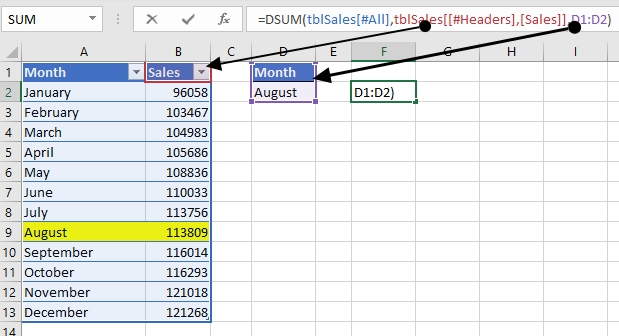• A1: B13–represents the Database
• E1–represents the field name of the column containing the data we want to return
• D1: D2–represents our criteria area

Above steps will also work for all below database functions

```=DMIN(A1:B13,B1,D1:D2)
=DAVERAGE(A1:B13,B1,D1:D2)
=DGET(A1:B13,B1,D1:D2)
=DPRODUCT(A1:B13,B1,D1:D2)
=DMAX(A1:B13,B1,D1:D2)```

# USE OF SLICERS

Slicers are a powerful way of filtering data. For them to work, the data has to be formatted as a table.

To convert a range to a table, select the whole range and press Ctrl+T.

Under table tools, click Insert Slicer, click the criteria field and press OK.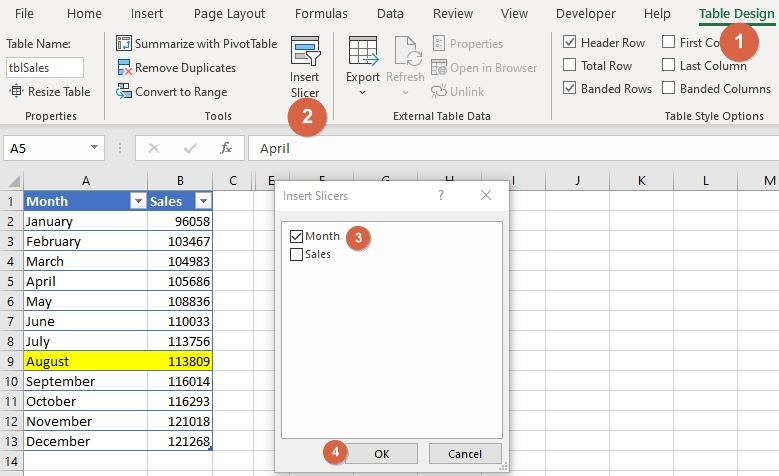Select the Month required.

# NUMEROUS OTHERS

Next time you feel stuck with VLOOKUP as the only option for looking up numbers, remember Excel offers us an embarrassment of Lookup-option riches.

Check out these comments in a Linkedin Group. Contains some overlooked functions like SUMQ, GETPIVOTDATA, SUMX2PY2, TRIMMEAN among others all of which can be used to look up numbers

RELATED ARTICLES

7 OVERLOOKED USES OF EXCEL MOD FUNCTION

7 ALTERNATIVES TO NESTED IF FUNCTION

7 WAYS TO USE EXCEL REPT FUNCTION

EXCEL DSUM FUNCTION

### RECOMMENDED BOOKS

EXCEL OUT OF BOX CREATIVITY BOOKS